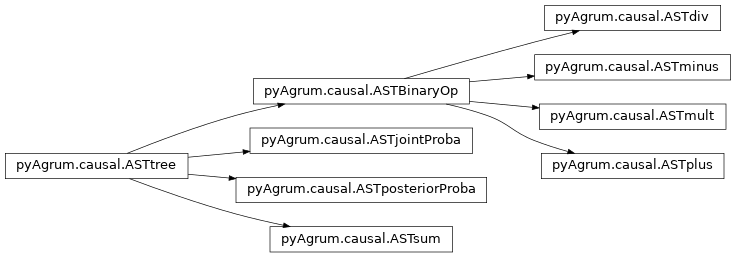# Abstract Syntax Tree for Do-Calculus¶

The pyCausal package compute every causal query into an Abstract Syntax Tree (CausalFormula) that represents the exact computations to be done in order to answer to the probabilistic causal query.

The different types of node in an CausalFormula are presented below and are organized as a hierarchy of classes from pyAgrum.causal.ASTtree.## Internal node structure¶

class pyAgrum.causal.ASTtree(type: str, verbose=False)

Represents a generic node for the CausalFormula. The type of the node will be registered in a string.

Parameters: type – the type of the node (will be specified in concrete children classes.
copy() → pyAgrum.causal._doAST.ASTtree

Copy an CausalFormula tree

Returns: the new causal tree
toLatex(nameOccur: Optional[Dict[str, int]] = None) → str

Create a LaTeX representation of a ASTtree

Returns: the LaTeX string
type

return: the type of the node

class pyAgrum.causal.ASTBinaryOp(type: str, op1: pyAgrum.causal._doAST.ASTtree, op2: pyAgrum.causal._doAST.ASTtree)

Represents a generic binary node for the CausalFormula. The op1 and op2 are the two operands of the class.

Parameters: type – the type of the node (will be specified in concrete children classes op1 – left operand op2 – right operand
copy() → pyAgrum.causal._doAST.ASTtree

Copy an CausalFormula tree

Returns: the new causal tree
op1

return: the left operand

op2

return: the right operand

toLatex(nameOccur: Optional[Dict[str, int]] = None) → str

Create a LaTeX representation of a ASTtree

Returns: the LaTeX string
type

return: the type of the node

## Basic Binary Operations¶

class pyAgrum.causal.ASTplus(op1: pyAgrum.causal._doAST.ASTtree, op2: pyAgrum.causal._doAST.ASTtree)

Represents the sum of 2 causal.ASTtree

Parameters: op1 – first operand op2 – second operand
copy() → pyAgrum.causal._doAST.ASTtree

Copy an CausalFormula tree

Returns: the new CausalFormula tree
op1

return: the left operand

op2

return: the right operand

toLatex(nameOccur: Optional[Dict[str, int]] = None) → str

Create a LaTeX representation of a ASTtree

Returns: the LaTeX string
type

return: the type of the node

class pyAgrum.causal.ASTminus(op1: pyAgrum.causal._doAST.ASTtree, op2: pyAgrum.causal._doAST.ASTtree)

Represents the substraction of 2 causal.ASTtree

Parameters: op1 – first operand op2 – second operand
copy() → pyAgrum.causal._doAST.ASTtree

Copy an CausalFormula tree

Returns: the new CausalFormula tree
op1

return: the left operand

op2

return: the right operand

toLatex(nameOccur: Optional[Dict[str, int]] = None) → str

Create a LaTeX representation of a ASTtree

Returns: the LaTeX string
type

return: the type of the node

class pyAgrum.causal.ASTdiv(op1: pyAgrum.causal._doAST.ASTtree, op2: pyAgrum.causal._doAST.ASTtree)

Represents the division of 2 causal.ASTtree

Parameters: op1 – first operand op2 – second operand
copy() → pyAgrum.causal._doAST.ASTtree

Copy an CausalFormula tree

Returns: the new CausalFormula tree
op1

return: the left operand

op2

return: the right operand

toLatex(nameOccur: Optional[Dict[str, int]] = None) → str

Create a LaTeX representation of a ASTtree

Returns: the LaTeX string
type

return: the type of the node

class pyAgrum.causal.ASTmult(op1: pyAgrum.causal._doAST.ASTtree, op2: pyAgrum.causal._doAST.ASTtree)

Represents the multiplication of 2 causal.ASTtree

Parameters: op1 – first operand op2 – second operand
copy() → pyAgrum.causal._doAST.ASTtree

Copy an CausalFormula tree

Returns: the new CausalFormula tree
op1

return: the left operand

op2

return: the right operand

toLatex(nameOccur: Optional[Dict[str, int]] = None) → str

Create a LaTeX representation of a ASTtree

Returns: the LaTeX string
type

return: the type of the node

## Complex operations¶

class pyAgrum.causal.ASTsum(var: List[str], term: pyAgrum.causal._doAST.ASTtree)

Represents a sum over a variable of a causal.ASTtree.

Parameters: var – name of the variable term – the tree to be evaluated
copy() → pyAgrum.causal._doAST.ASTtree

Copy an CausalFormula tree

Returns: the new CausalFormula tree
eval(contextual_bn: pyAgrum.pyAgrum.BayesNet) → pyAgrum.pyAgrum.Potential

Evaluation of the sum

Parameters: contextual_bn – BN where to infer the value of the sum
toLatex(nameOccur: Optional[Dict[str, int]] = None) → str

Create a LaTeX representation of a ASTtree

Returns: the LaTeX string
type

return: the type of the node

class pyAgrum.causal.ASTjointProba(varNames: Set[str])

Represent a joint probability in the base observational part of the causal.CausalModel

Parameters: varNames – a set of variable names
copy() → pyAgrum.causal._doAST.ASTtree

Copy an CausalFormula tree

Returns: the new CausalFormula tree
toLatex(nameOccur: Optional[Dict[str, int]] = None) → str

Create a LaTeX representation of a ASTtree

Returns: the LaTeX string
type

return: the type of the node

varNames

return: the set of names of var

class pyAgrum.causal.ASTposteriorProba(bn: pyAgrum.pyAgrum.BayesNet, vars: Set[str], knw: Set[str])

Represent a conditional probability $$P_{bn}(vars|knw)$$ that can be computed by an inference in a BN.

Parameters: bn – the pyAgrum:pyAgrum.BayesNet vars – a set of variable names (in the BN) knw – a set of variable names (in the BN)
bn

return: bn in $$P_{bn}(vars|knw)$$

copy() → pyAgrum.causal._doAST.ASTtree

Copy an CausalFormula tree

Returns: the new CausalFormula tree
knw

return: knw in $$P_{bn}(vars|knw)$$

toLatex(nameOccur: Optional[Dict[str, int]] = None) → str

Create a LaTeX representation of a ASTtree

Returns: the LaTeX string
type

return: the type of the node

vars

return: vars in $$P_{bn}(vars|knw)$$